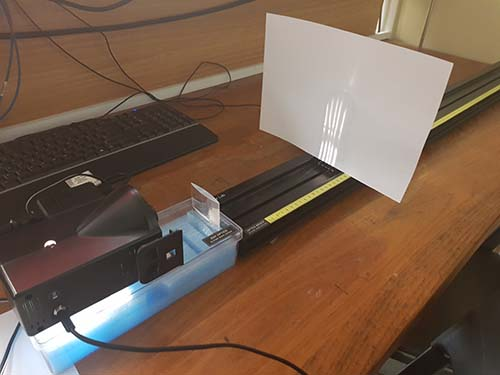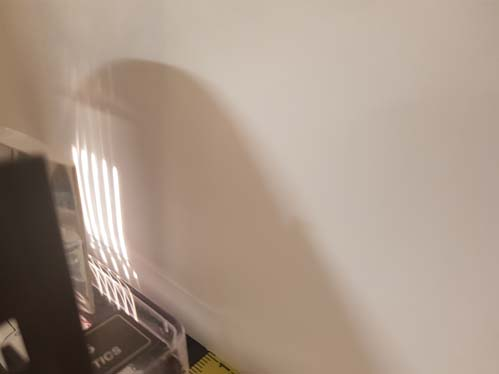Home   Thin Lens

Thin Lens
Virtual Lab - Thin Lenses

# Virtual Lab - Thin Lenses

A lens has two focal points, one either side of the lens. The focal length f is the distance between the lens and the focal point. f is
positive for a converging lens
negative for a diverging lens.

The object distance s is the distance between the lens and the object. s is (nearly) always positive.
The image distance s’ is the distance between the lens and the image.
s’ is
positive if the image is on the other side of the lens from the light source (real)
negative if the image is on the same side of the lens as the light source (virtual)

The thin-lens equation relates the object distance and the image distance with the focal length: Similarly the lateral magnification of the image is given by; The image formed by a converging lens depends on the position of the object.
The image is described as being
real or virtual
erect or inverted
magnified or diminishedExperimental setup, showing the object, the lens, and the image screen.The image screen, showing an in/out of focus image

To focus the image to determine the focal length,
click increase ... length button until image is in focus.

Image screen:

Reset screen length

Measurments
Current Object length is cm
Current Image screen length is cm

 Measurment Object Length (cm) Image Screen Legnth (cm) 1 2 3 4

MassForce